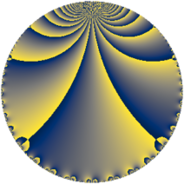# Properties

 Label 64.4.bLevel $64$ Weight $4$ Character orbit 64.b Rep. character $\chi_{64}(33,\cdot)$ Character field $\Q$ Dimension $6$ Newform subspaces $2$ Sturm bound $32$ Trace bound $1$

# Related objects

## Defining parameters

 Level: $$N$$ $$=$$ $$64 = 2^{6}$$ Weight: $$k$$ $$=$$ $$4$$ Character orbit: $$[\chi]$$ $$=$$ 64.b (of order $$2$$ and degree $$1$$) Character conductor: $$\operatorname{cond}(\chi)$$ $$=$$ $$8$$ Character field: $$\Q$$ Newform subspaces: $$2$$ Sturm bound: $$32$$ Trace bound: $$1$$ Distinguishing $$T_p$$: $$3$$

## Dimensions

The following table gives the dimensions of various subspaces of $$M_{4}(64, [\chi])$$.

Total New Old
Modular forms 30 6 24
Cusp forms 18 6 12
Eisenstein series 12 0 12

## Trace form

 $$6 q - 54 q^{9} + O(q^{10})$$ $$6 q - 54 q^{9} + 156 q^{17} - 18 q^{25} - 696 q^{33} + 828 q^{41} + 1014 q^{49} - 1368 q^{57} - 2304 q^{65} + 876 q^{73} + 6942 q^{81} - 2676 q^{89} - 8772 q^{97} + O(q^{100})$$

## Decomposition of $$S_{4}^{\mathrm{new}}(64, [\chi])$$ into newform subspaces

Label Dim $A$ Field CM Traces $q$-expansion
$a_{2}$ $a_{3}$ $a_{5}$ $a_{7}$
64.4.b.a $2$ $3.776$ $$\Q(\sqrt{-1})$$ $$\Q(\sqrt{-2})$$ $$0$$ $$0$$ $$0$$ $$0$$ $$q+5iq^{3}-73q^{9}+9iq^{11}+90q^{17}+\cdots$$
64.4.b.b $4$ $3.776$ $$\Q(\zeta_{12})$$ None $$0$$ $$0$$ $$0$$ $$0$$ $$q+\zeta_{12}q^{3}-\zeta_{12}^{2}q^{5}+\zeta_{12}^{3}q^{7}+23q^{9}+\cdots$$

## Decomposition of $$S_{4}^{\mathrm{old}}(64, [\chi])$$ into lower level spaces

$$S_{4}^{\mathrm{old}}(64, [\chi]) \cong$$ $$S_{4}^{\mathrm{new}}(8, [\chi])$$$$^{\oplus 4}$$$$\oplus$$$$S_{4}^{\mathrm{new}}(32, [\chi])$$$$^{\oplus 2}$$